# 「干货总结」程序员必知必会的十大排序算法bigsai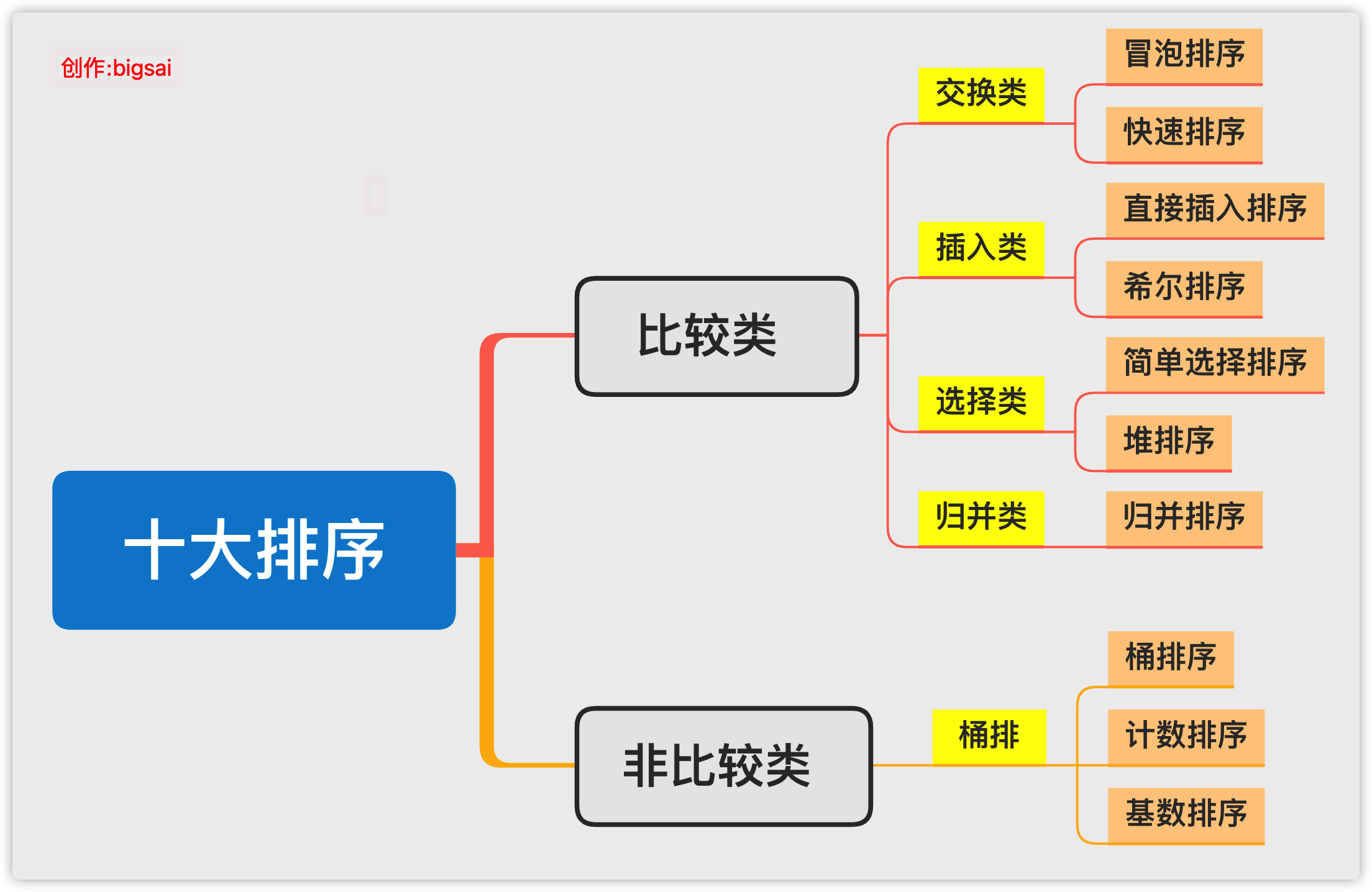# ﻿

﻿

## 绪论

﻿

﻿

﻿

﻿

• 非比较类的有桶排序、基数排序、计数排序。也有很多人将排序归纳为8大排序，那就是因为基数排序、计数排序是建立在桶排序之上或者是一种特殊的桶排序，但是基数排序和计数排序有它特有的特征，所以在这里就将他们归纳为10种经典排序算法。而比较类排序也可分为

• 比较类排序也有更细致的分法，有基于交换的、基于插入的、基于选择的、基于归并的，更细致的可以看下面的脑图。

﻿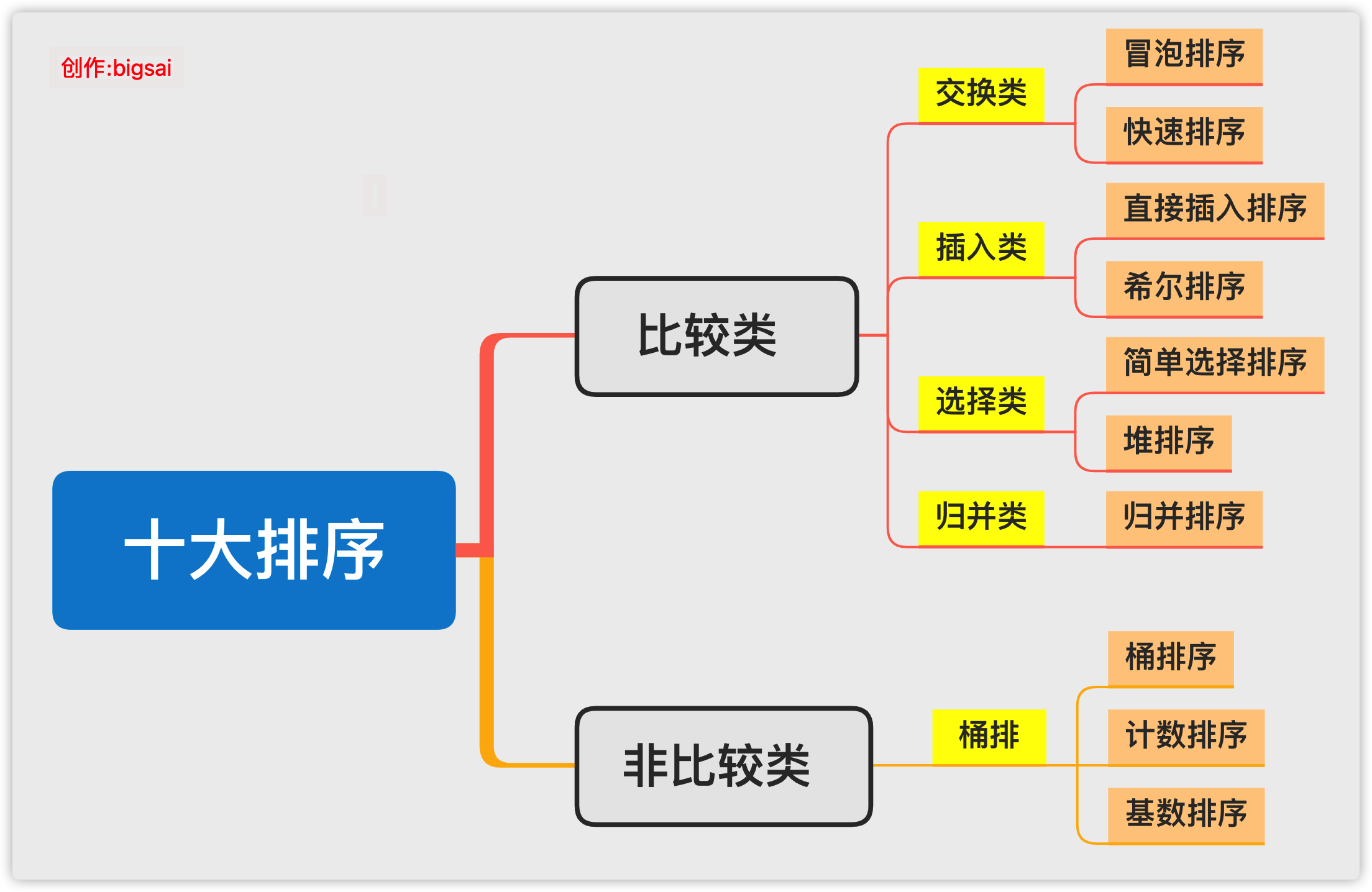﻿

## 交换类

### 冒泡排序

﻿

﻿

• 从第一个元素开始往后遍历，每到一个位置判断是否比后面的元素大，如果比后面元素大，那么就交换两者大小，然后继续向后，这样的话进行一轮之后就可以保证最大的那个数被交换交换到最末的位置可以确定

• 第二次同样从开始起向后判断着前进，如果当前位置比后面一个位置更大的那么就和他后面的那个数交换。但是有点注意的是，这次并不需要判断到最后，只需要判断到倒数第二个位置就行(因为第一次我们已经确定最大的在倒数第一，这次的目的是确定倒数第二)

• 同理，后面的遍历长度每次减一，直到第一个元素使得整个元素有序。

﻿

﻿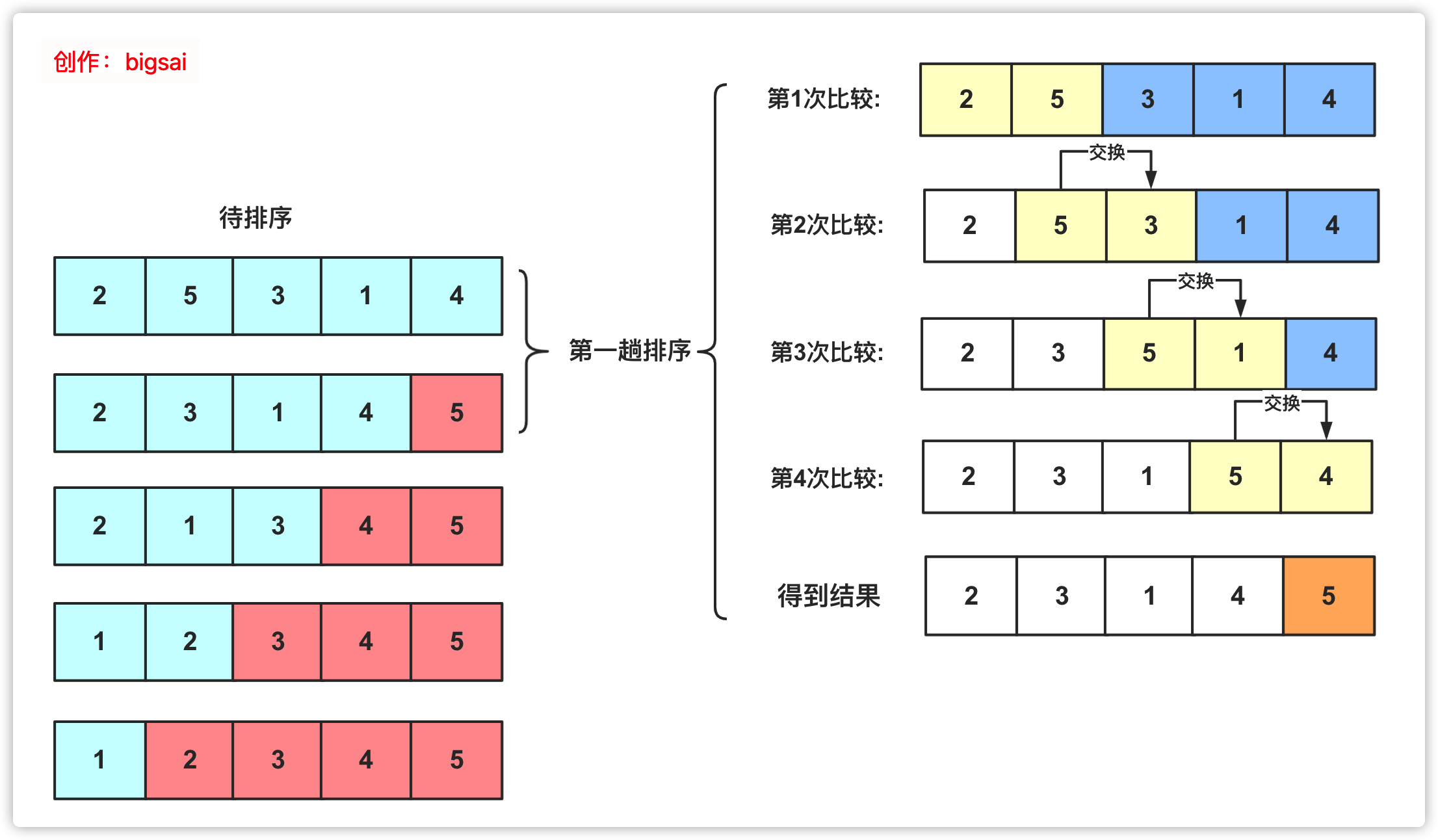﻿

﻿

public void  maopaosort(int[] a) {  // TODO Auto-generated method stub  for(int i=a.length-1;i>=0;i--)  {    for(int j=0;j<i;j++)    {      if(a[j]>a[j+1])      {        int team=a[j];        a[j]=a[j+1];        a[j+1]=team;      }    }  }}

﻿

### 快速排序

• 快排需要将序列变成两个部分，就是序列左边全部小于一个数，*序列右面全部大于一个数*，然后利用递归的思想再将左序列当成一个完整的序列再进行排序，同样把序列的右侧也当成一个完整的序列进行排序。

• 其中这个数在这个序列中是可以随机取的，可以取最左边，可以取最右边，当然也可以取随机数。但是通常不优化情况我们取最左边的那个数。

﻿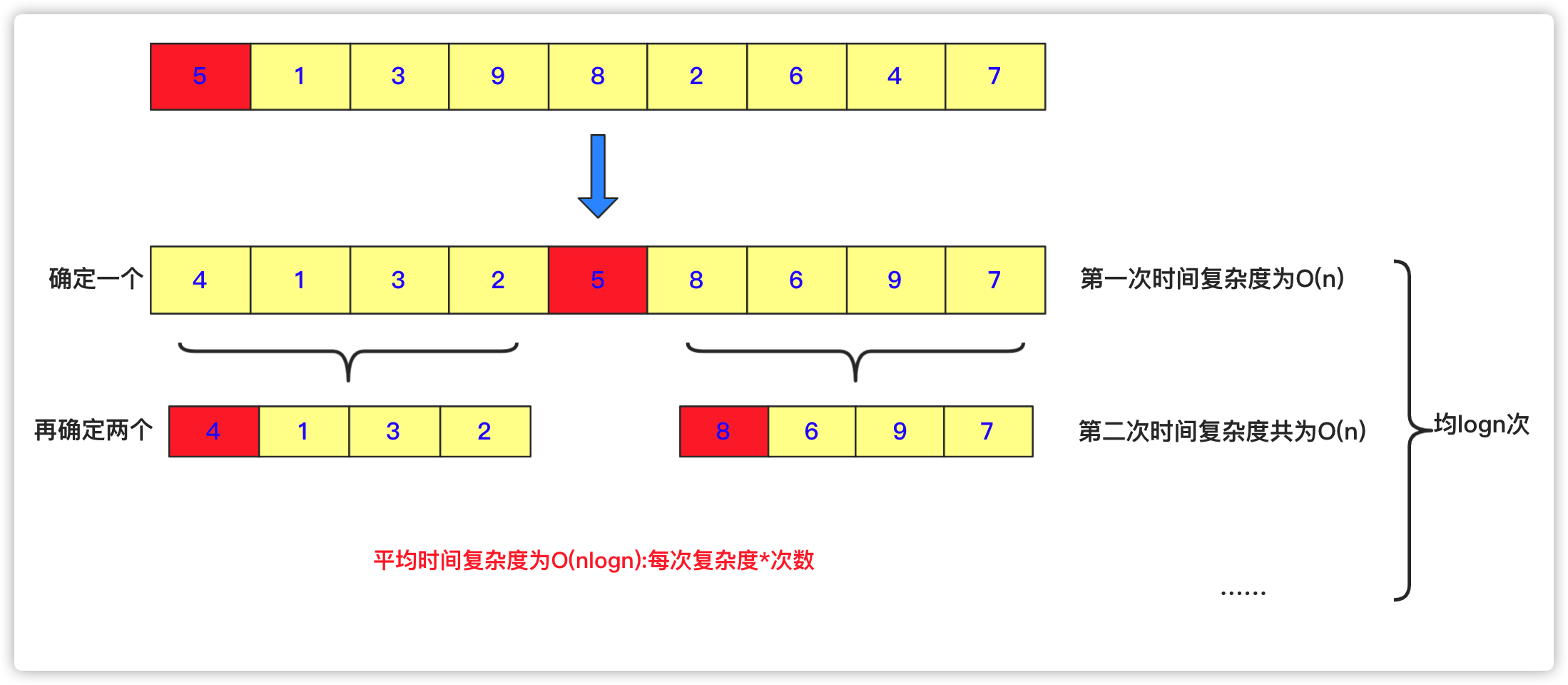﻿

﻿

public void quicksort(int [] a,int left,int right){  int low=left;  int high=right;  //下面两句的顺序一定不能混，否则会产生数组越界！！！very important！！！  if(low>high)//作为判断是否截止条件    return;  int k=a[low];//额外空间k，取最左侧的一个作为衡量，最后要求左侧都比它小，右侧都比它大。  while(low<high)//这一轮要求把左侧小于a[low],右侧大于a[low]。  {    while(low<high&&a[high]>=k)//右侧找到第一个小于k的停止    {      high--;    }    //这样就找到第一个比它小的了    a[low]=a[high];//放到low位置    while(low<high&&a[low]<=k)//在low往右找到第一个大于k的，放到右侧a[high]位置    {      low++;    }    a[high]=a[low];			  }  a[low]=k;//赋值然后左右递归分治求之  quicksort(a, left, low-1);  quicksort(a, low+1, right);		}

## 插入类排序

### 直接插入排序

﻿

﻿

• 选取当前位置(当前位置前面已经有序) 目标就是将当前位置数据插入到前面合适位置。

• 向前枚举或者二分查找，找到待插入的位置。

• 移动数组，赋值交换，达到插入效果。

﻿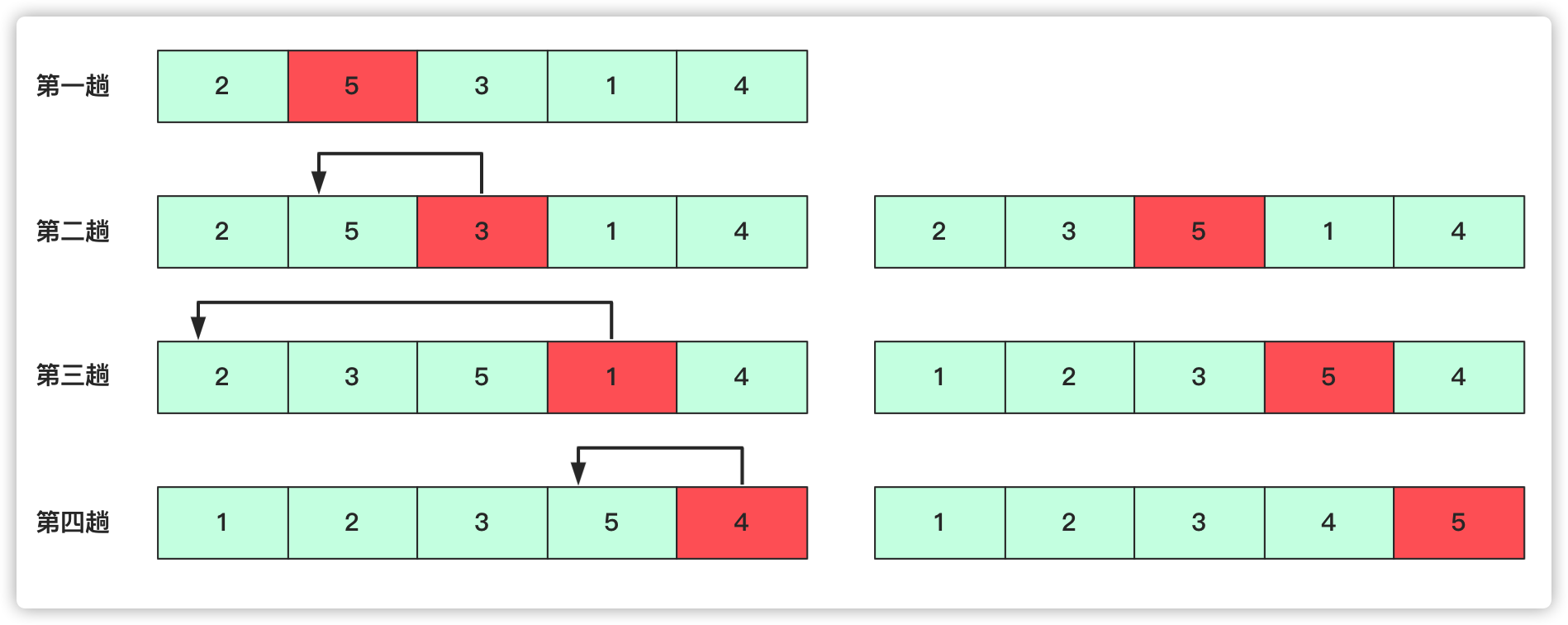﻿

﻿

public void insertsort (int a[]){  int team=0;  for(int i=1;i<a.length;i++)  {    System.out.println(Arrays.toString(a));    team=a[i];    for(int j=i-1;j>=0;j--)    {      if(a[j]>team)      {        a[j+1]=a[j];        a[j]=team;	      }	      else {        break;      }    }  }	}

### 希尔排序

﻿

﻿

﻿

﻿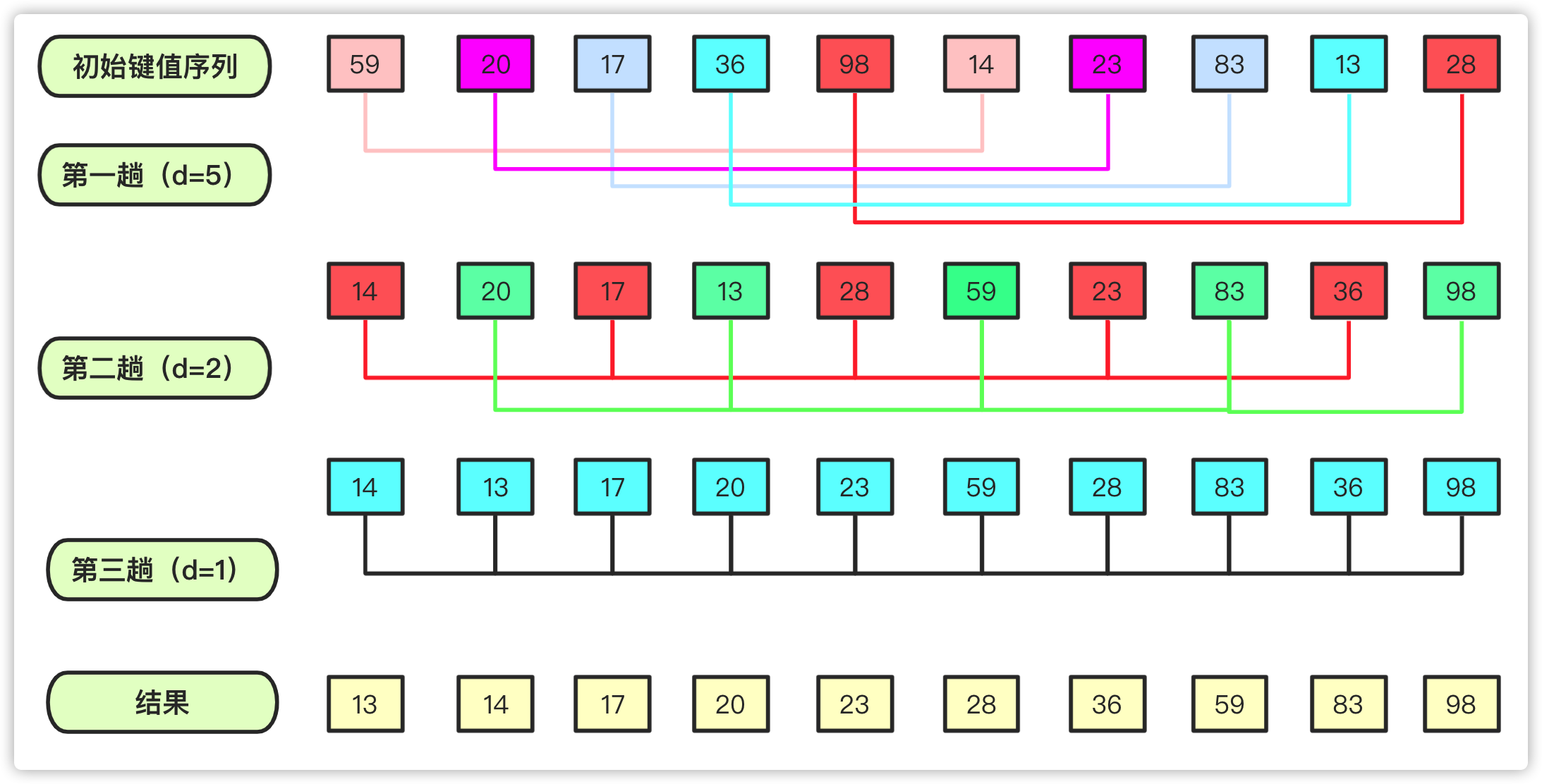﻿

﻿

public void shellsort (int a[]){  int d=a.length;  int team=0;//临时变量  for(;d>=1;d/=2)//共分成d组    for(int i=d;i<a.length;i++)//到那个元素就看这个元素在的那个组即可    {      team=a[i];      for(int j=i-d;j>=0;j-=d)      {				        if(a[j]>team)        {          a[j+d]=a[j];          a[j]=team;	        }        else {          break;        }      }    }	}

﻿

## 选择类排序

### 简单选择排序

﻿

﻿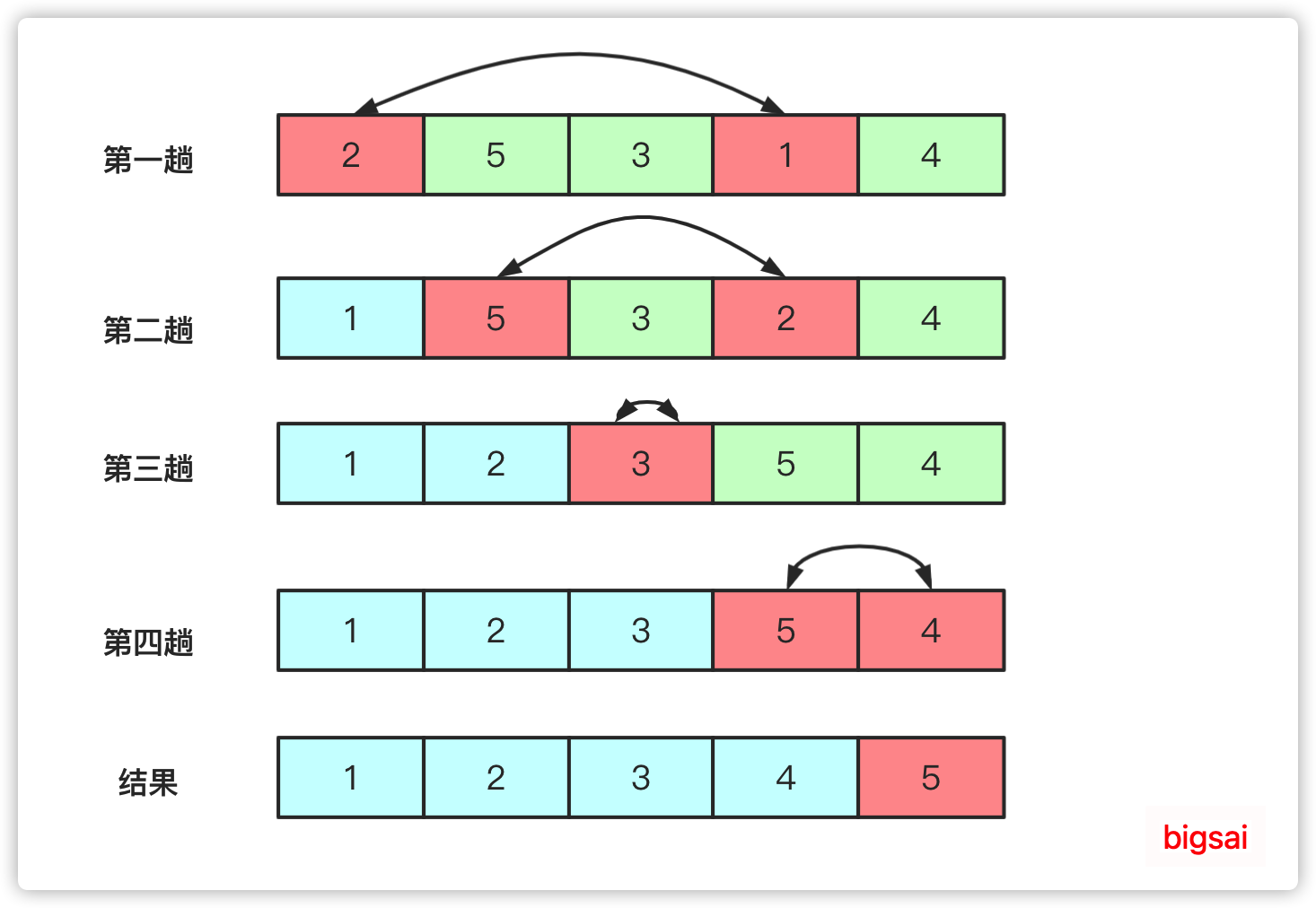﻿

﻿

public void selectSort(int[] arr) {  for (int i = 0; i < arr.length - 1; i++) {    int min = i; // 最小位置    for (int j = i + 1; j < arr.length; j++) {      if (arr[j] < arr[min]) {        min = j; // 更换最小位置      }    }    if (min != i) {      swap(arr, i, min); // 与第i个位置进行交换    }  }}private void swap(int[] arr, int i, int j) {  int temp = arr[i];  arr[i] = arr[j];  arr[j] = temp;}

﻿

### 堆排序

• 如果所有节点大于孩子节点值，那么这个堆叫做大根堆，堆的最大值在根节点。

• 如果所有节点小于孩子节点值，那么这个堆叫做小根堆，堆的最小值在根节点。

﻿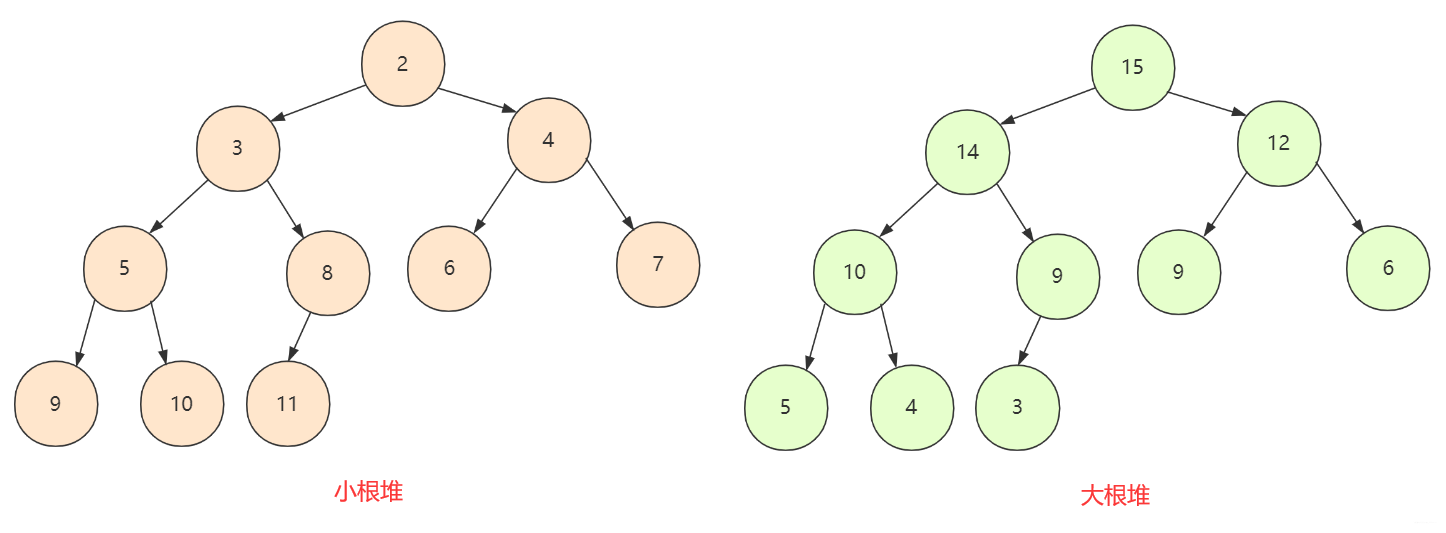﻿

①从第一个非叶子节点开始判断交换下移(shiftDown)，使得当前节点和子孩子能够保持堆的性质

②但是普通节点替换可能没问题，对如果交换打破子孩子堆结构性质，那么就要重新下移(shiftDown)被交换的节点一直到停止。

﻿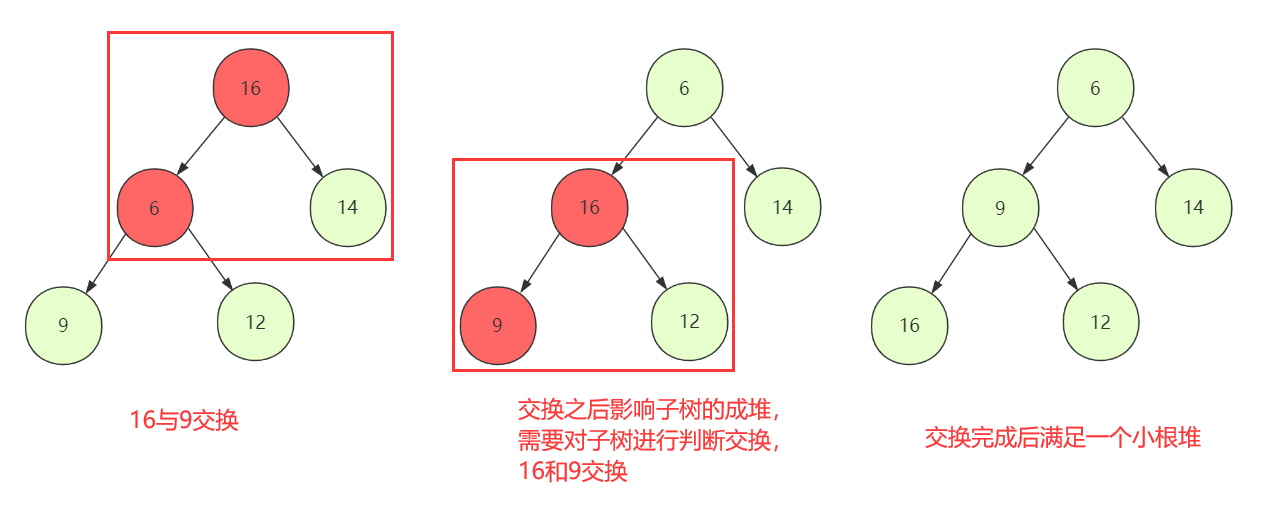﻿

①所以索性把最后一个元素放到第一位。这样只需要判断交换下移(shiftDown）,不过需要注意此时整个堆的大小已经发生了变化，我们在逻辑上不会使用被抛弃的位置，所以在设计函数的时候需要附带一个堆大小的参数。

②重复以上操作，一直堆中所有元素都被取得停止。

﻿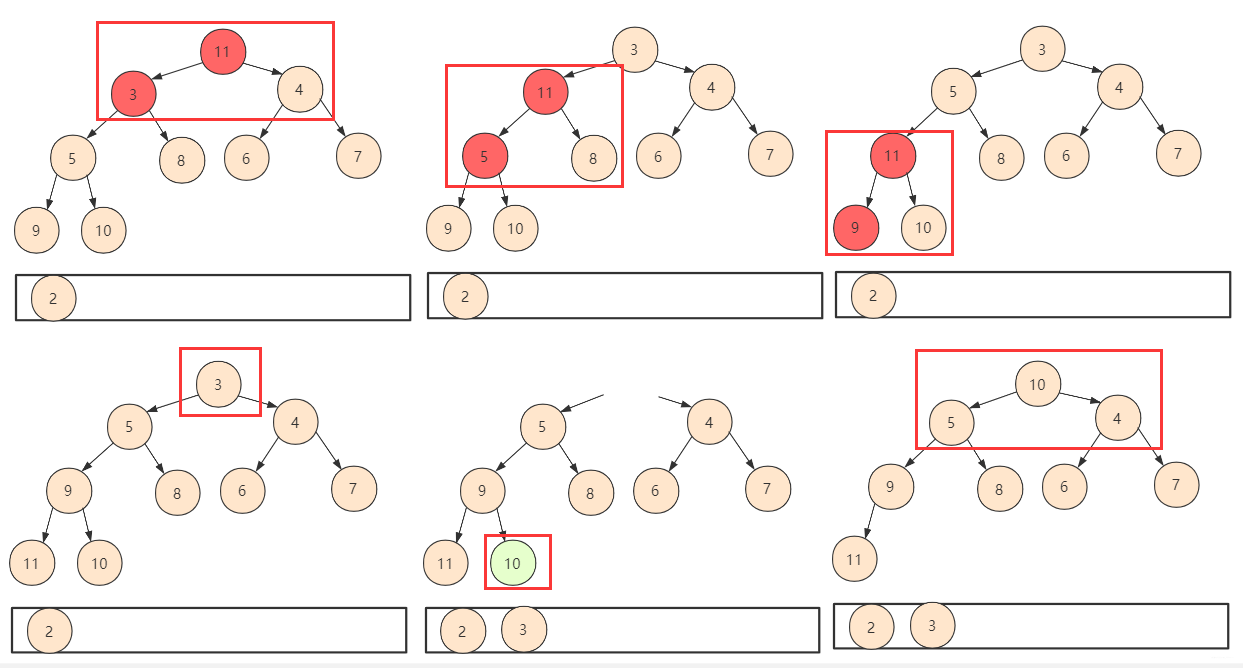﻿

static void swap(int arr[],int m,int n){  int team=arr[m];  arr[m]=arr[n];  arr[n]=team;}//下移交换 把当前节点有效变换成一个堆(小根)static void shiftDown(int arr[],int index,int len)//0 号位置不用{  int leftchild=index*2+1;//左孩子  int rightchild=index*2+2;//右孩子  if(leftchild>=len)    return;  else if(rightchild<len&&arr[rightchild]<arr[index]&&arr[rightchild]<arr[leftchild])//右孩子在范围内并且应该交换  {    swap(arr, index, rightchild);//交换节点值    shiftDown(arr, rightchild, len);//可能会对孩子节点的堆有影响，向下重构  }  else if(arr[leftchild]<arr[index])//交换左孩子  {    swap(arr, index, leftchild);    shiftDown(arr, leftchild, len);  }}//将数组创建成堆static void creatHeap(int arr[]){  for(int i=arr.length/2;i>=0;i--)  {    shiftDown(arr, i,arr.length);  }}static void heapSort(int arr[]){  System.out.println("原始数组为         ："+Arrays.toString(arr));  int val[]=new int[arr.length]; //临时储存结果  //step1建堆  creatHeap(arr);  System.out.println("建堆后的序列为  ："+Arrays.toString(arr));  //step2 进行n次取值建堆，每次取堆顶元素放到val数组中，最终结果即为一个递增排序的序列  for(int i=0;i<arr.length;i++)  {    val[i]=arr;//将堆顶放入结果中    arr=arr[arr.length-1-i];//删除堆顶元素，将末尾元素放到堆顶    shiftDown(arr, 0, arr.length-i);//将这个堆调整为合法的小根堆，注意(逻辑上的)长度有变化  }  //数值克隆复制  for(int i=0;i<arr.length;i++)  {    arr[i]=val[i];  }  System.out.println("堆排序后的序列为:"+Arrays.toString(arr));}

## 归并类排序

﻿

### 归并排序

﻿

• 第一次：整串先进行划分成一个一个单独，第一次是将序列中(1 2 3 4 5 6---)两两归并成有序，归并完(xx xx xx xx----)这样局部有序的序列。

• 第二次就是两两归并成若干四个(1 2 3 4 5 6 7 8 ----)每个小局部是有序的

• 就这样一直到最后这个串串只剩一个，然而这个耗费的总次数logn。每次操作的时间复杂的又是O(n)。所以总共的时间复杂度为O(nlogn).

﻿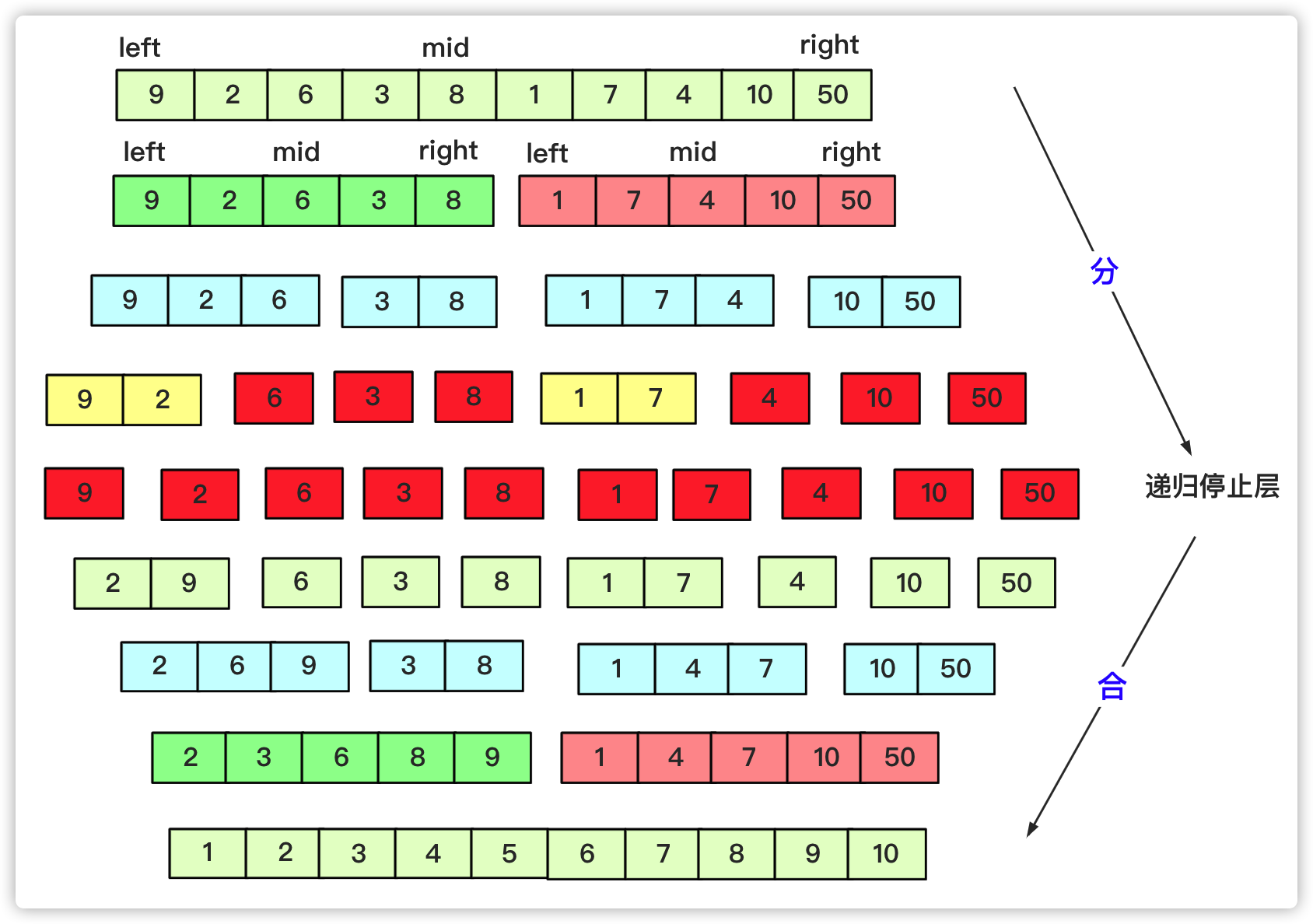﻿

﻿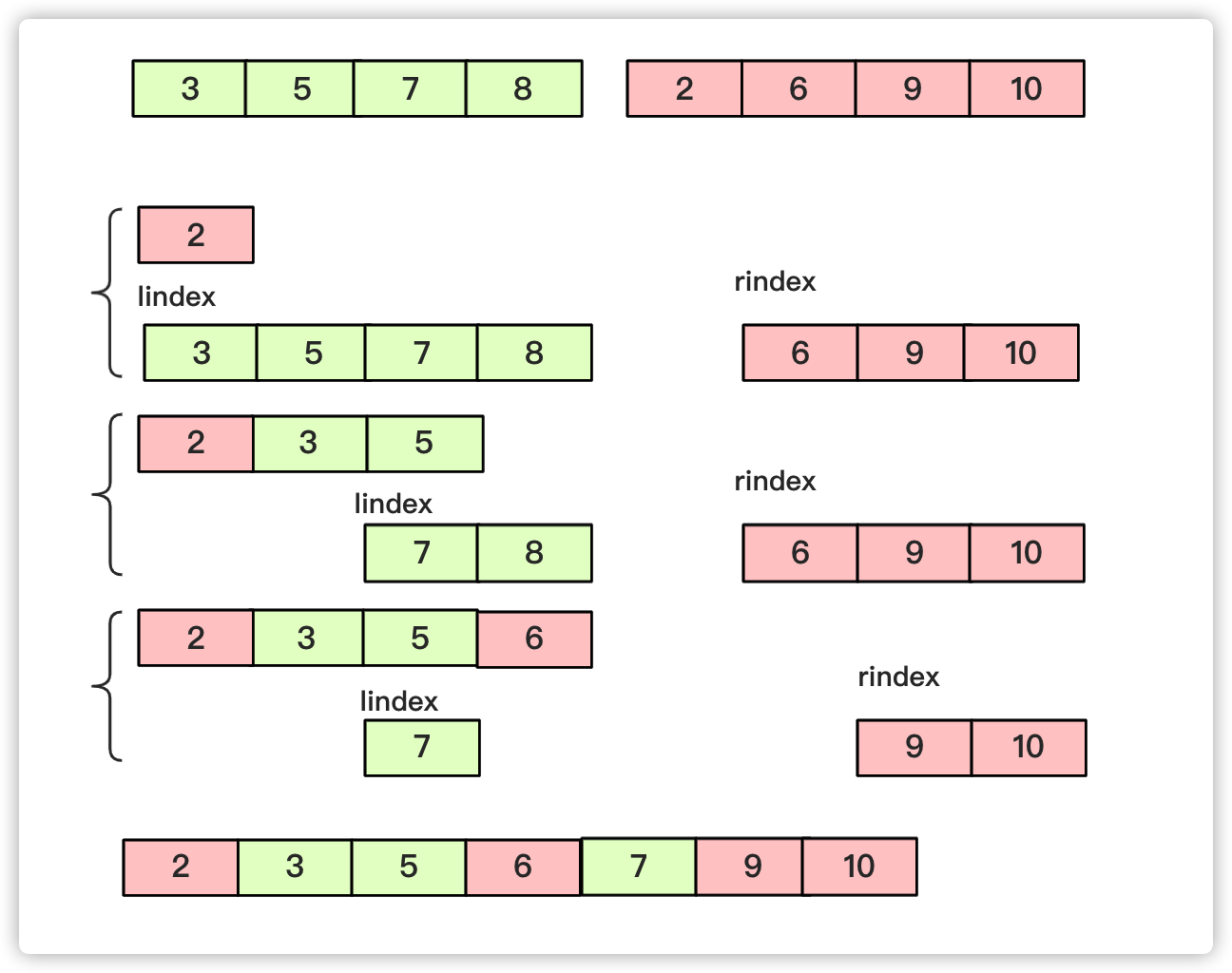﻿

﻿

private static void mergesort(int[] array, int left, int right) {  int mid=(left+right)/2;  if(left<right)  {    mergesort(array, left, mid);    mergesort(array, mid+1, right);    merge(array, left,mid, right);  }}private static void merge(int[] array, int l, int mid, int r) {  int lindex=l;int rindex=mid+1;  int team[]=new int[r-l+1];  int teamindex=0;  while (lindex<=mid&&rindex<=r) {//先左右比较合并    if(array[lindex]<=array[rindex])    {      team[teamindex++]=array[lindex++];    }    else {				      team[teamindex++]=array[rindex++];    }  }  while(lindex<=mid)//当一个越界后剩余按序列添加即可  {    team[teamindex++]=array[lindex++];  }  while(rindex<=r)  {    team[teamindex++]=array[rindex++];  }	  for(int i=0;i<teamindex;i++)  {    array[l+i]=team[i];  }}

## 桶类排序

### 桶排序

• 桶：若干个桶，说明此类排序将数据放入若干个桶中。

• 桶：每个桶有容量，桶是有一定容积的容器，所以每个桶中可能有多个元素。

• 桶：从整体来看，整个排序更希望桶能够更匀称，即既不溢出(太多)又不太少。

﻿

﻿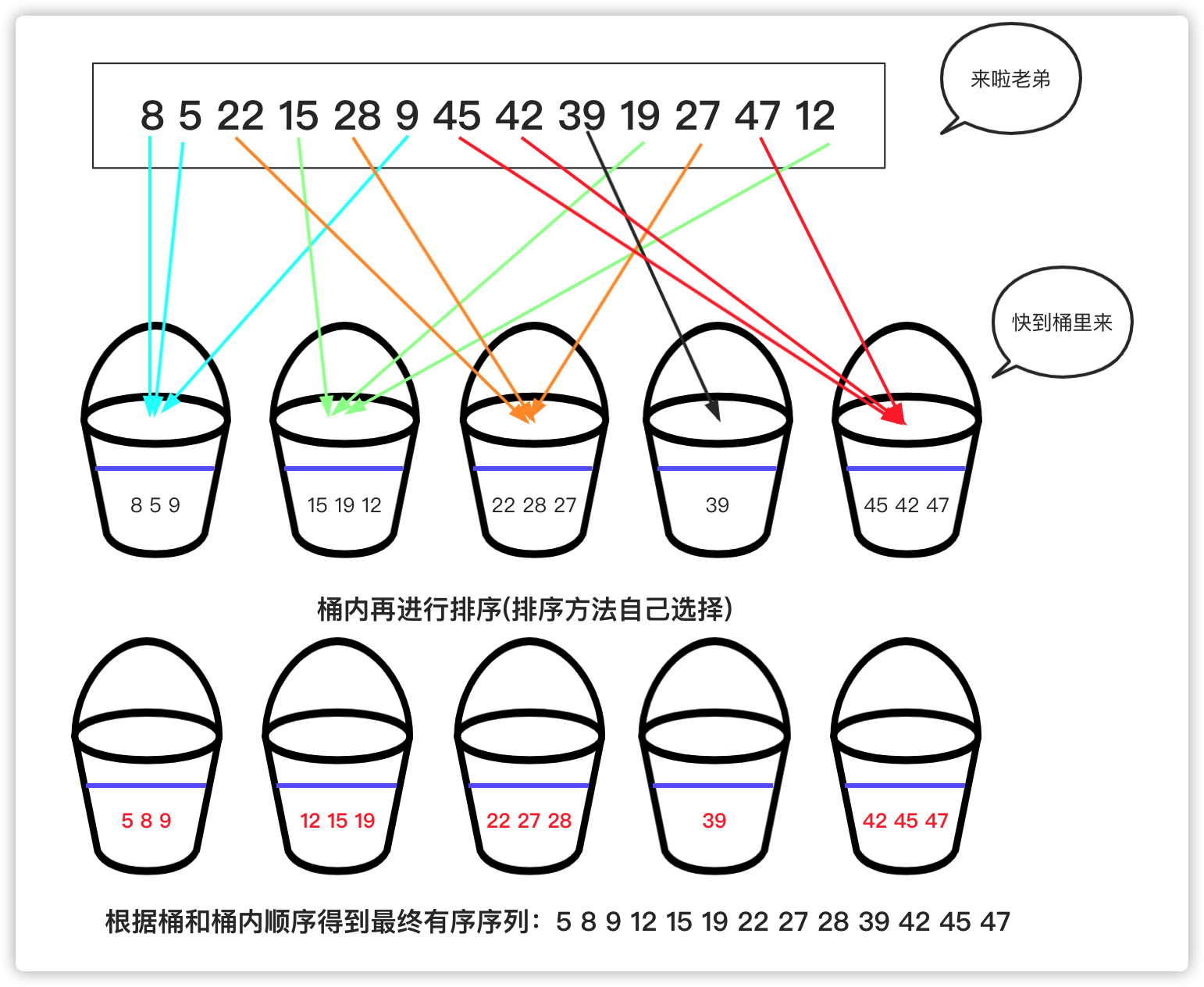﻿

﻿

import java.util.ArrayList;import java.util.List;//微信公众号：bigsaipublic class bucketSort {	public static void main(String[] args) {		int a[]= {1,8,7,44,42,46,38,34,33,17,15,16,27,28,24};		List[] buckets=new ArrayList;		for(int i=0;i<buckets.length;i++)//初始化		{			buckets[i]=new ArrayList<Integer>();		}		for(int i=0;i<a.length;i++)//将待排序序列放入对应桶中		{			int index=a[i]/10;//对应的桶号			buckets[index].add(a[i]);		}		for(int i=0;i<buckets.length;i++)//每个桶内进行排序(使用系统自带快排)		{			buckets[i].sort(null);			for(int j=0;j<buckets[i].size();j++)//顺便打印输出			{				System.out.print(buckets[i].get(j)+" ");			}		}		}}

### 计数排序

﻿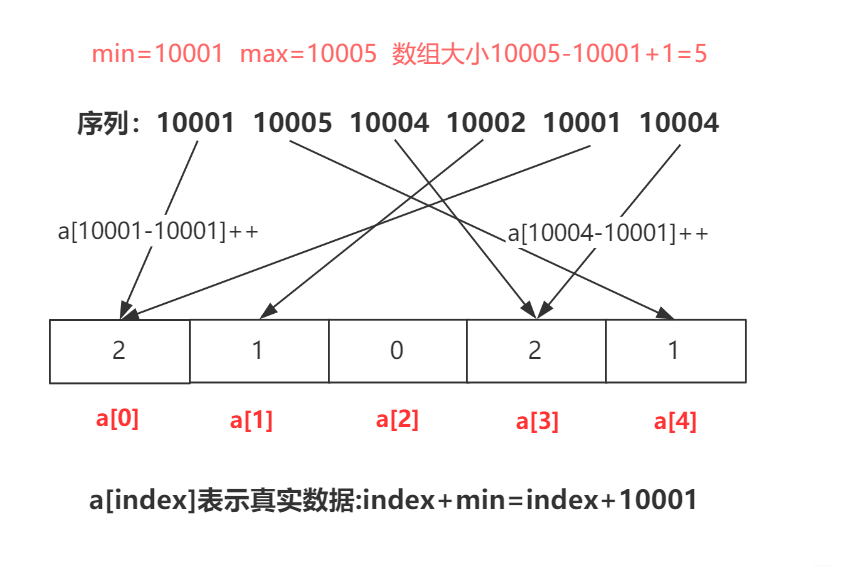﻿

public static void countSort(int a[]){  int min=Integer.MAX_VALUE;int max=Integer.MIN_VALUE;  for(int i=0;i<a.length;i++)//找到max和min  {    if(a[i]<min)       min=a[i];    if(a[i]>max)      max=a[i];  }  int count[]=new int[max-min+1];//对元素进行计数  for(int i=0;i<a.length;i++)  {    count[a[i]-min]++;  }  //排序取值  int index=0;  for(int i=0;i<count.length;i++)  {    while (count[i]-->0) {      a[index++]=i+min;//有min才是真正值    }  }}

### 基数排序

﻿﻿

﻿

﻿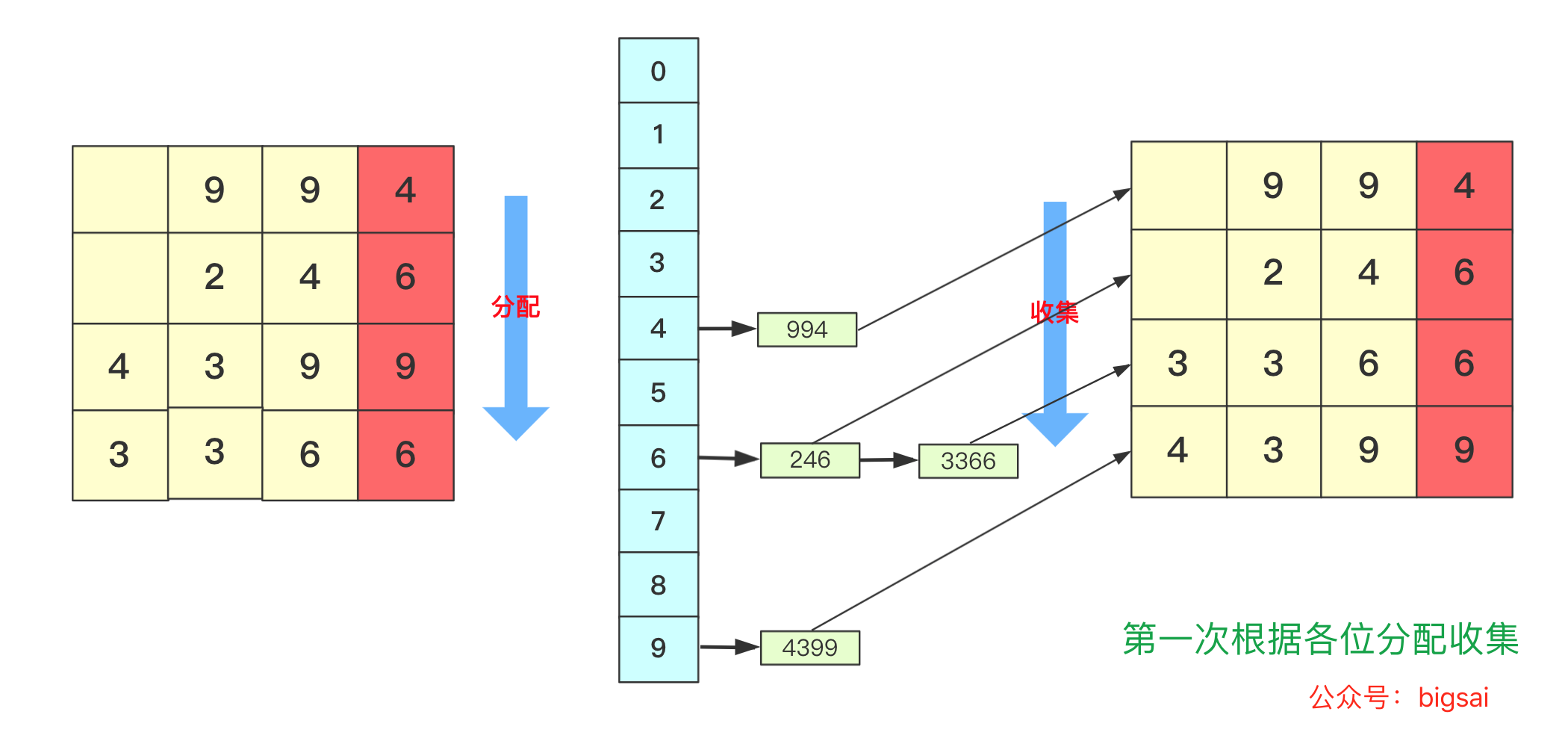﻿

﻿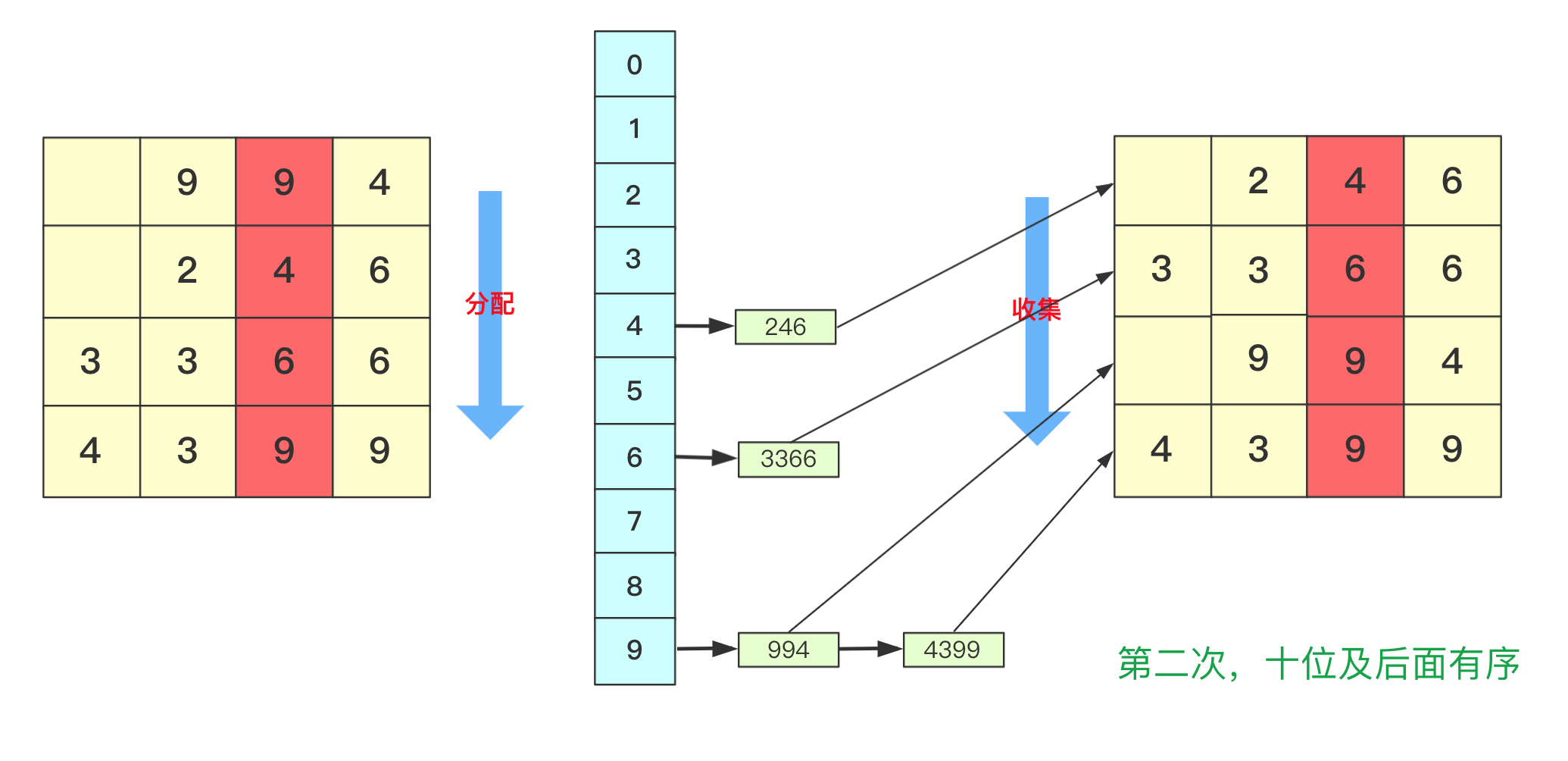﻿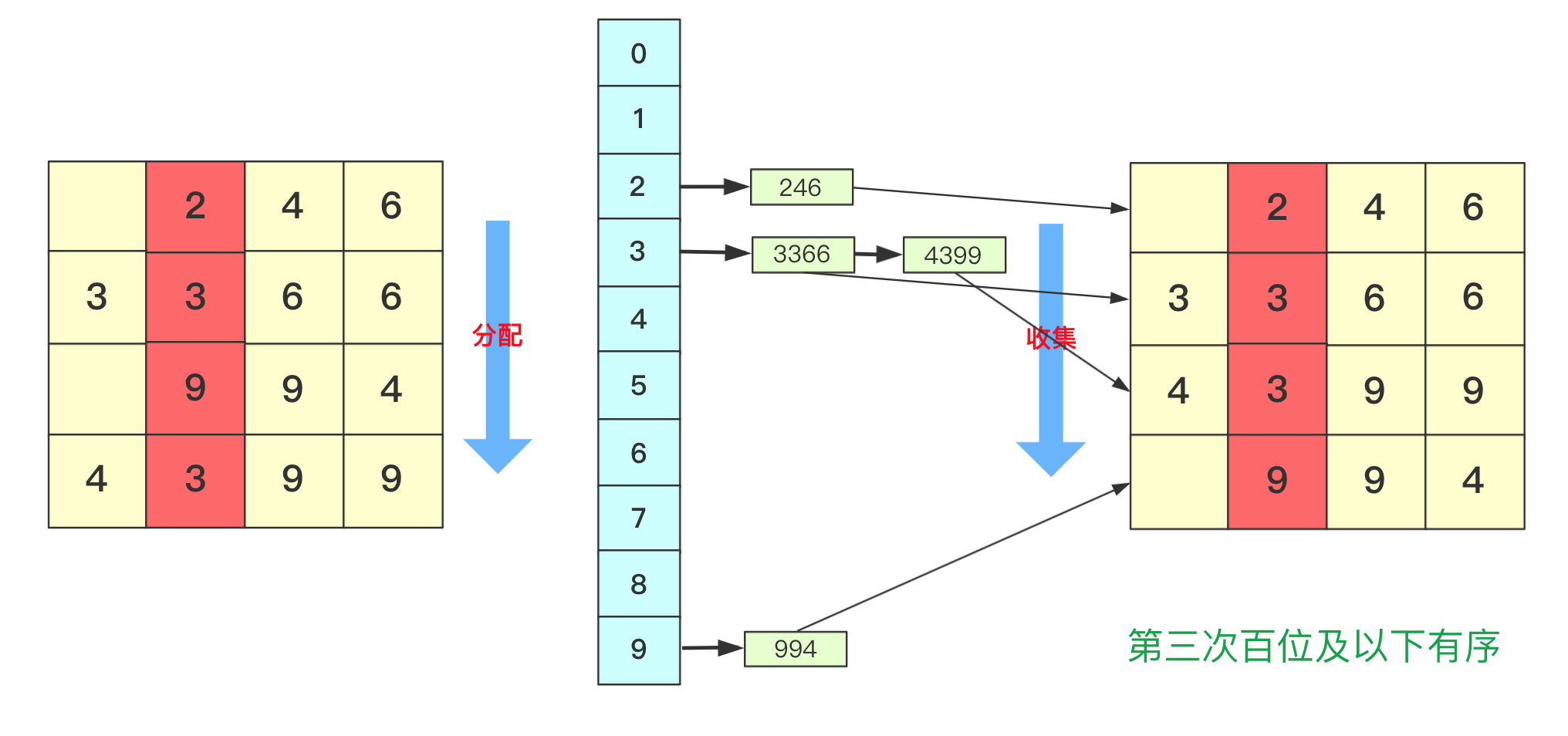﻿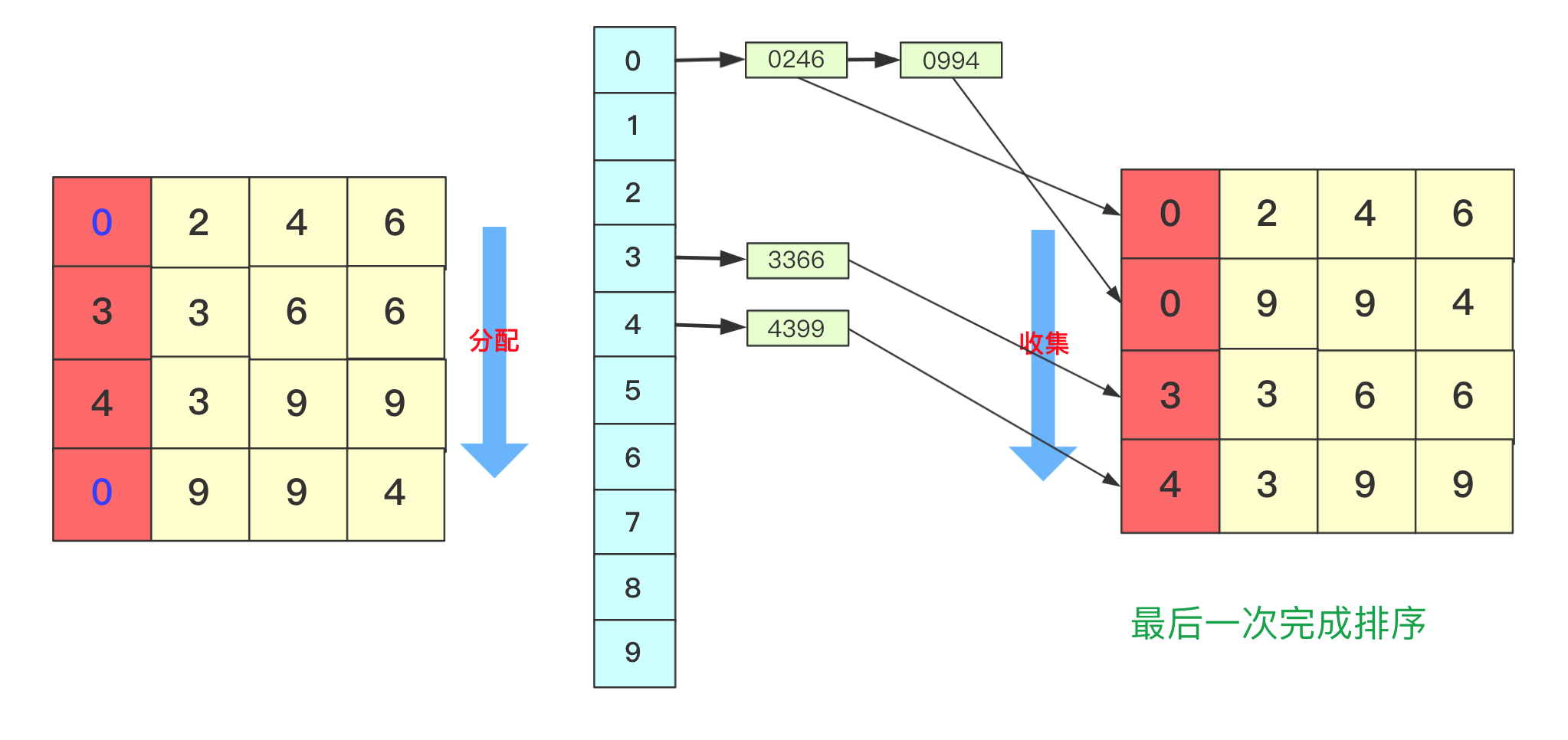﻿

static void radixSort(int[] arr)//int 类型 从右往左{  List<Integer>bucket[]=new ArrayList;  for(int i=0;i<10;i++)  {    bucket[i]=new ArrayList<Integer>();  }  //找到最大值  int max=0;//假设都是正数  for(int i=0;i<arr.length;i++)  {    if(arr[i]>max)      max=arr[i];  }  int divideNum=1;//1 10 100 100……用来求对应位的数字  while (max>0) {//max 和num 控制    for(int num:arr)    {      bucket[(num/divideNum)%10].add(num);//分配 将对应位置的数字放到对应bucket中    }    divideNum*=10;    max/=10;    int idx=0;    //收集 重新捡起数据    for(List<Integer>list:bucket)    {      for(int num:list)      {        arr[idx++]=num;      }      list.clear();//收集完需要清空留下次继续使用    }  }}

﻿

## 结语

﻿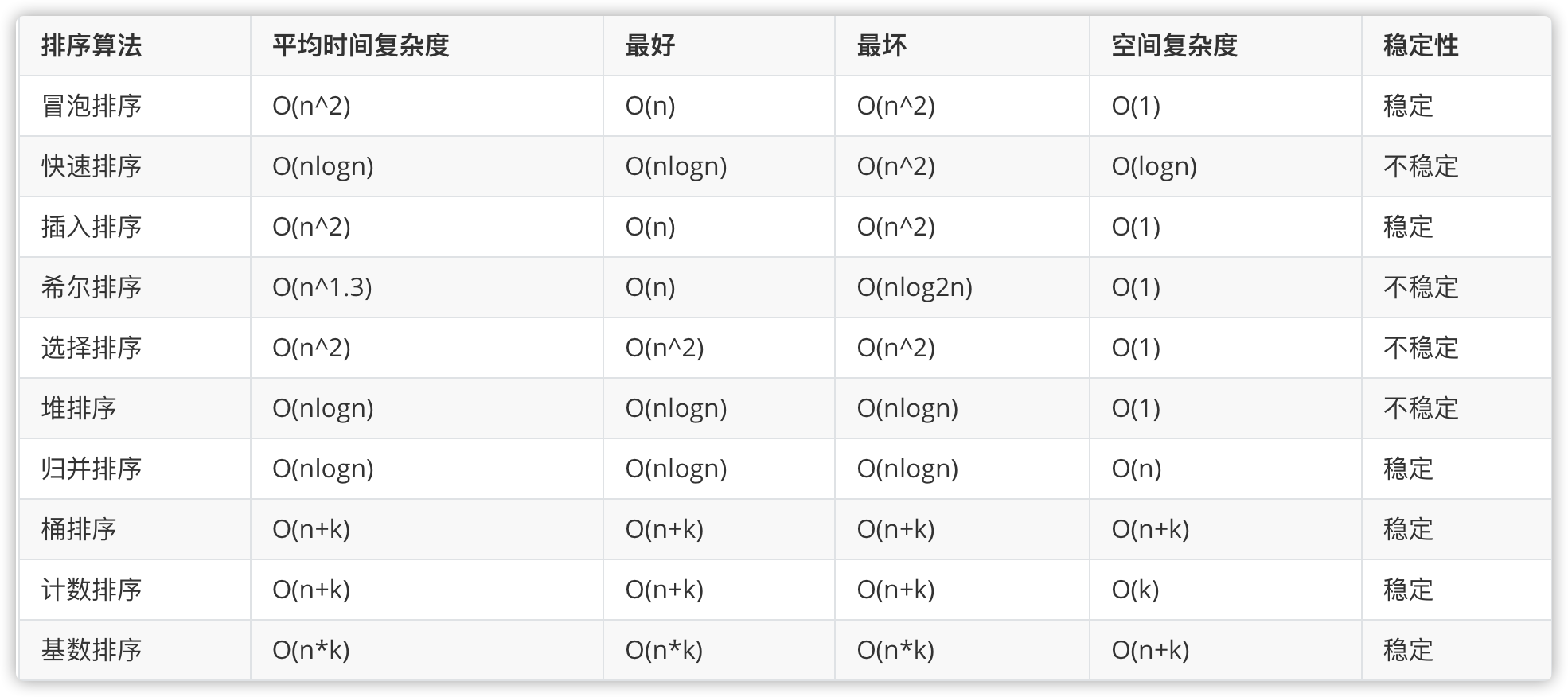﻿

1. 点赞、收藏、关注支持一下， 您的肯定是我在info创作的源源动力。

2. 微信搜索「bigsai」，关注我的公众号，不仅免费送你电子书，我还会第一时间在公众号分享知识技术。加我还可拉你进力扣打卡群一起打卡LeetCode。﻿## 评论